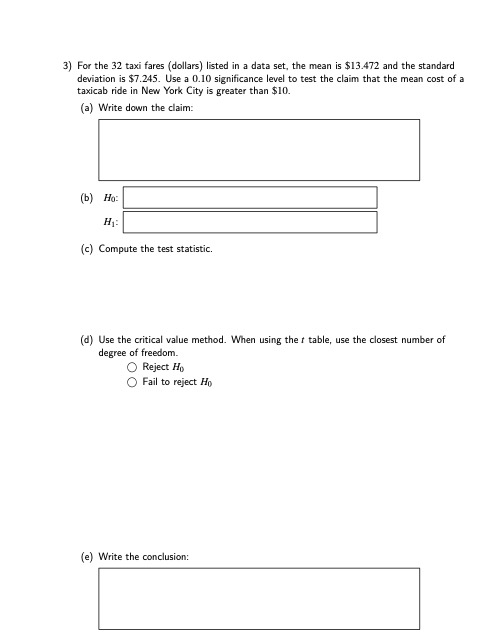# Question 3) For the 32 taxi fares (dollars) listed in a data set, the mean is \$13.472 and the standard deviation is \$7.245. Use a 0.10 significance level to test the claim that the mean cost of a taxicab ride in New York City is greater than \$10. (a) Write down the claim: (b) Ho: H: (c) Compute the test statistic, (d) Use the critical value method. When using the table, use the closest number of degree of freedom Reject H Fail to reject H. (e) Write the conclusion:WXGNK0 The Asker · Probability and StatisticsTranscribed Image Text: 3) For the 32 taxi fares (dollars) listed in a data set, the mean is \$13.472 and the standard deviation is \$7.245. Use a 0.10 significance level to test the claim that the mean cost of a taxicab ride in New York City is greater than \$10. (a) Write down the claim: (b) Ho: H: (c) Compute the test statistic, (d) Use the critical value method. When using the table, use the closest number of degree of freedom Reject H Fail to reject H. (e) Write the conclusion:
More
Transcribed Image Text: 3) For the 32 taxi fares (dollars) listed in a data set, the mean is \$13.472 and the standard deviation is \$7.245. Use a 0.10 significance level to test the claim that the mean cost of a taxicab ride in New York City is greater than \$10. (a) Write down the claim: (b) Ho: H: (c) Compute the test statistic, (d) Use the critical value method. When using the table, use the closest number of degree of freedom Reject H Fail to reject H. (e) Write the conclusion: